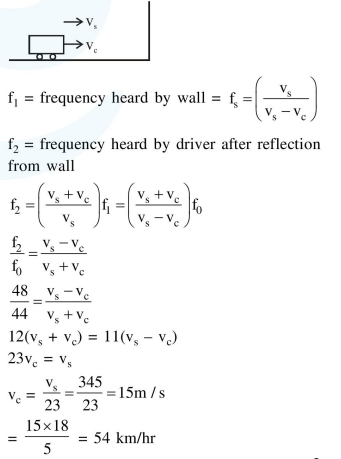# A driver in a car, approaching a vertical wall notices

Question:

A driver in a car, approaching a vertical wall notices that the frequency of his car horn, has changed from $440 \mathrm{~Hz}$ to $480 \mathrm{~Hz}$, when it gets reflected from the wall. If the speed of sound in air is $345 \mathrm{~m} / \mathrm{s}$, then the speed of the car is

1. $36 \mathrm{~km} / \mathrm{hr}$

2. $24 \mathrm{~km} / \mathrm{hr}$

3. $18 \mathrm{~km} / \mathrm{hr}$

4. $54 \mathrm{~km} / \mathrm{hr}$

Correct Option: , 4

Solution: